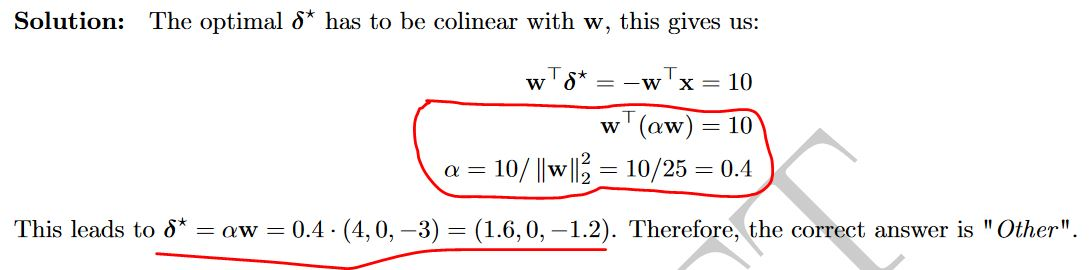### Question 14 exam 2020

Hi,
we have w and x given, so that their product is -10. Then we wanna find the optimal delta, which is the distance from x to the hyperplane, defined by w. So i know that delta has to be collinear, and opposed in direction, wrt w. However, I don't get the steps in red here:Thank you so much!

Hi,
$\alpha$ is a scalar so the formula is the same as $\alpha * w.T . w$ which is the same as $\alpha / ||w||^2$

Page 1 of 1